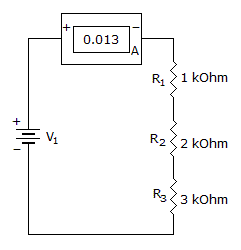# Electronics - Series Circuits - Discussion

### Discussion :: Series Circuits - General Questions (Q.No.5)

5.

What is the dc source voltage?[A]. 78 V [B]. 39 V [C]. 13 V [D]. 0 V

Explanation:

No answer description available for this question.

 Sundar said: (Oct 21, 2010) Ohm's Law: V = IR Given I = 0.013A; R = R1+R2+R3 = (1+2+3) Kohm = 6 Kohm = 6000 ohm. Therefore, V = 0.013 * 6000 = 78 volts.

 Mintu said: (Jul 9, 2012) V = IR. Total R = 1+2+3 = 6Kohm. V = .013*6Kohm = 78V.

 Nivetha said: (Nov 4, 2013) Given that, I = 13mA. R1 = 1K, R2 = 2K, R3 = 3K. Then V = IR. V = 13*(1+2+3). V = 13*6 = 78 Volts. NOTE: Here milli amps & Kilo ohms gets cancelled.

 Saptarshi Dutta said: (Dec 9, 2013) V = IxRt. Rt = R1+R2+R3 = 6000 ohm. Hence, V = 0.013X6000 = 78 Volts.

 Manvendra Singh said: (Aug 26, 2015) V = IR. R = R1+R2+R3 = 1+2+3 = 6 kohm V = 13*6 = 78 volts.

 Sri said: (Jul 4, 2016) What is the resistance of 0.013A source box?

 Hatu said: (Jul 4, 2016) As per my understanding, 0.013A source resistance is not known. Hence answer cannot be predicted.

 Neeraj said: (Nov 19, 2016) @Hatu @Sri. Since it is an ammeter, And Assuming it as an ideal Ammeter with net resistance 0.